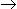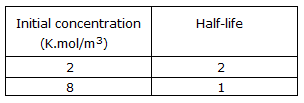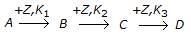# Chemical Engineering - Chemical Reaction Engineering

### Exercise :: Chemical Reaction Engineering - Section 9

1.

The following half life data are available for the irreversible liquid phase reaction Aproducts.The overall order of reaction is

 A. 0.5 B. 1 C. 1.5 D. 2

Explanation:

No answer description available for this question. Let us discuss.

2.

A non-catalytic chemical reaction of the typeis called a __________ reaction.

 A. parallel B. series C. series-parallel D. none of these

Explanation:

No answer description available for this question. Let us discuss.

3.

The unit of frequency factor in Arhenious equation is

 A. same as that of rate constant. B. same as that of activation energy. C. dimensionless. D. none of these.

Explanation:

No answer description available for this question. Let us discuss.

4.

With increase in the order of reaction (for all positive reaction orders), the ratio of the volume of mixed reactor to the volume of plug flow reactor (for identical feed composition, flow rate and conversion)

 A. increases B. decreases C. remains same D. increases linearly

Explanation:

No answer description available for this question. Let us discuss.

5.

Reaction of benzene with chlorine gas to produce tri-chlorobenzene exemplifies a/an __________ reaction.

 A. elementary B. parallel C. consecutive D. none of these